Excursion into Lagrange Interpolation.

Two points in the plane determine a line, and therefore in coordinate geometry, the coordinates for two points determine the equation of a line. If we consider the case where the two points have different first coordinates, we can find a linear function the graph of which is the line determined by the two points. The method of Joseph Louis Lagrange, (1736-1813), called LaGrange interpolation resolves a comparable problem for determining a polynomial function of degree n the graph of which passes through n+1 points in the plane when no two of these points have the same first coordinate.

In this excursion we'll look at the simplest cases when n = 1 and 2 to get a sense of how this method works, and leave the more general examples for the exercises. With a small number of points, this method is sometimes used to find a polynomial of low degree for estimating other information about a less well-known function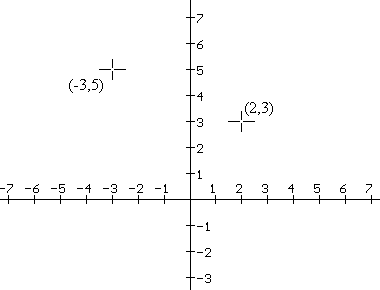Example 1. We'll find the linear function that has its graph pass through two given points as an example of Lagrange's method. [Of course there are many methods you have studied for solving this problem. We are interested here on a method that will generalize for more points and polynomial functions of higher degree.] For this example, suppose the line passes through (2,3) and (-3,5). See Figures 1 and 2.

For Lagrange's method we suppose the linear function has the form

f (X) = Y = R (X-2) + S (X+3).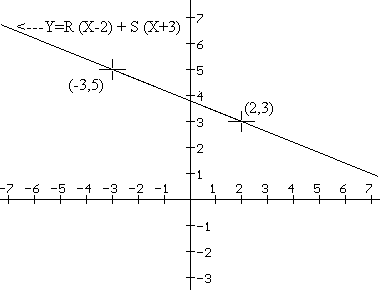Then using the coordinate information we replace the X and Y in the equation with X=2 and Y=3, giving us the equation 3= R(2-2) + S(2+3) or 3 = 5S, so S = 3/5. Similarly we can use X=-3, and Y=5, so 5 = -5R and thus R = -1. So the equation

f (X) =Y = -1(X-2) + 3/5 (X+3)

should work. You can check without much trouble that both the given points lie on the line this function determines. Notice how using factors of (X-2) and (X+3) in the form of the linear function made it easy to find the values for R and S.

Example 2. We'll find the quadratic function describing a parabola that passes through three given points using Lagrange's method. For this example, suppose the parabola passes through (2,3), (-3,5), and (1,-7). See Figure 3. For Lagrange's method we suppose the quadratic function has the form

p(X) = Y = R (X-2)(X-1) + S (X+3)(X-1) + T (X-2)(X+3).

Using the coordinate information from the three given points we can determine R, S, and T. First we use X=2 andY=3 to see that 3 = 5S, so S = 3/5. Similarly using X = -3 and Y = 5 we have 5 = 20 R, so R = 1/4 and with X = 1 and Y = -7 we find that -7 = -4T, so T = 7/4. So the equation for the quadratic function is

p(X)=Y = 1/4 (X-2)(X-1) + 3/5 (X+3)(X-1) + 7/4(X-2)(X+3).

You can check this quadratic function does determine a parabola through the three given points. Notice how using factors of (X-2)(X-1), (X+3)(X-1), and (X-2)(X+3) in the form of the quadratic functions made it easy to find the values for R, S, and T.

1. Describe the key feature of the form of the linear function in Example 1 that enabled us to solve the problem.
1. Suppose the problem in example 1 had points (2,1) and (-1,2). Use Lagrange's technique with f (X) = Y = R (X-a) + S (X-c) to find the equation for the appropriate linear function.
2. Suppose the problem in example 1 had points (a,b) and (-c,d) with a?b. Use Lagrange's technique with f (X) = Y = R (X-a) + S (X-c) to show that the appropriate linear function is given by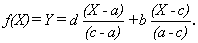3.
2. Describe the key feature of the form of the quadratic polynomila function in Example 2 that enabled us to solve the problem.
1. Suppose the problem in example 2 had points (1,2), (2,1), and (3,3). Use Lagrange's technique with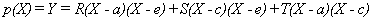to find the quadratic polynomial function with its graph passing through these three points.
2. Suppose the problem in example 2 had points (1,2), (2,1), and (3,0). These three points all lie on the same line. Use Lagrange's technique withto show that the graph of no quadratic function could pass through these three points. [Hint: Suppose that there was such a quadratic and work out the values for R, S, and T. Then expand the polynomial and notice that R+S+T=0.]
3. Suppose the problem in example 2 had points (a,b), (c,d), and (e,f) with a, c, and e all different numbers. Use Lagrange's technique withto show that the appropriate parabola has equation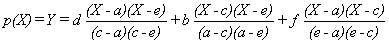.
3. Use Lagrange interpolation to find an appropriate function passing through the given points. Sketch a graph of this function based only on the given points and what you think the curve must be. Compare your sketch with the graph created by graphing technology.
1. A linear function passing through the points (-1,3) and (2,1).
2. A quadratic function passing through the points (-1,3), (0,2), and (2,1).
3. A cubic function passing through the points (-1,3), (0,2), (1,5), and (2,1).
4. A quartic (fourth degree) polynomial function passing through (-2,4), (-1,3), (0,2), (1,5), and (2,1).

5.
4. Finding a quadratic function that resembles other functions: By choosing three noncolinear points on any curve we can use Lagrange's interpolation to find a parabola that passes through those points. For each of the following functions find a parabola that passes through the graphs of the functions when at points with the indicated first coordinates. Use graphing technology to draw a sketch of the function and the quadratic function you find. Discuss how you might use the function you find to estimate the value of the given function.
1.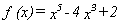; x = 0, 1, 2.
2.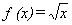; x = 0, 1, 4.
3.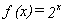; x = -1, 0, 1.
4.; x = 0, 1, 2.
5.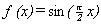; x = 0, 1, 2.
6.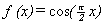; x = -1, 0, 1.

7.
5. Describe a general procedure for finding a polynomial function of degree n that passes through n+1 given points with distinct first coordinates.